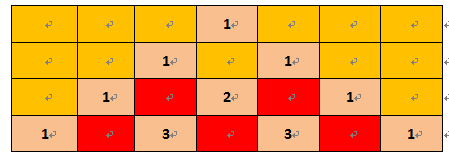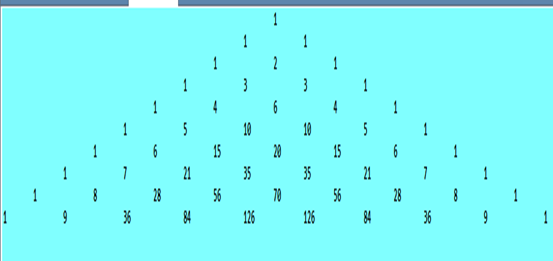• 不过在写出杨辉三角形的公式时，使用了六个变量，之后的学习中，尽量用少的变量达成效果。毕竟字符是有限的，得省着用，而且多个变量以为着过程的重复，不得不在for语句中装了3个while。 同时，知道了在运算过程中会...
今天的作业，是完成杨辉三角形的创作（虽然有点小歪^ v ^）
在创作过程中。我再一次温习了for语句的嵌套，并首次在for语句当中嵌套两个有先后执行顺序的for语句。不过在写出杨辉三角形的公式时，使用了六个变量，之后的学习中，尽量用少的变量达成效果。毕竟字符是有限的，得省着用，而且多个变量以为着过程的重复，不得不在for语句中装了3个while。
同时，知道了在运算过程中会变得很大的数值，一定要用double来定义而不是int，否则会计算不了，还查不出啥问题。
希望之后的学习能更加精益求精，减少出错，在缩减代码数量的前提下达成一样的效果。展开全文• ## 杨辉三角形

千次阅读 2015-05-05 20:25:40
在屏幕上打印出杨辉三角形。 1 1 1 1 2 1 1 3 3 1 1 4 6 4 1 1 5 10 10 5 1 根据上图可以得到2个公式： 1）每行第一个和最后一个为1 2）除上式得两个数外，其他数分别是上一行中前一列和同一列两数之和...
在屏幕上打印出杨辉三角形。

       1
1  1
1  2  1
1  3   3  1
1  4  6   4  1
1  5  10  10  5  1


根据上图可以得到2个公式：
1）每行的第一个和最后一个为1
2）除上式得两个数外，其他数分别是上一行中的前一列和同一列的两数之和。

#include<stdio.h>

int add(int x,int y);

int main()
{
int i,j,n=13;
printf("N=");
while(n>12)
scanf("%d",&n);

for(i=0;i<=n;i++)
{
for(j=0;j<24-2*i;j++)
printf(" ");
for(j=1;j<i+2;j++)
printf("%4d",add(i,j));
printf("\n");
}

return 0;
}

int add(int x,int y)
{
int z;
if((y==1)||(y==x+1))
return 1;
z=add(x-1,y-1)+add(x-1,y);

return z;
}

欢迎大家点击左上角的“关注”或右上角的“收藏”方便以后阅读。

为使本文得到斧正和提问，转载请注明出处： http://blog.csdn.net/nomasp
展开全文int add
• Java中杨辉三角形的制作 一.杨辉三角形解释： 杨辉三角形由数字排列，可以把它看做一个数字表，其基本特性是两侧数值均为1，其他位置的数值是其正上方的数字与左上角数值之和。编写程序，使用for循环输出包括10行...
Java中杨辉三角形的制作
一.杨辉三角形解释：
杨辉三角形由数字排列，可以把它看做一个数字表，其基本特性是两侧数值均为1，其他位置的数值是其正上方的数字与左上角数值之和。编写程序，使用for循环输出包括10行在内的杨辉三角形。 思路是创建一个整型二维数组，包含10个一维数组。使用双层循环，在外层循环中初始化每一个第二层数组的大小。在内层循环中，先将两侧的数组元素赋值为1，其他数值通过公式计算，然后输出数组元素。
二.示例图：三.源代码如下：
package test;

public class Test {
public static void main(String[] args) {
int m = 10, k = m * 2 - 1;
int[][] a = new int[m][k + 1];   //默认全部是0
// 行
for (int i = 0; i < a.length; i++) {
//列
for (int j = 0; j < k; j++) {
//将两侧全部置为1
if (j == k / 2 - i || j == k / 2 + i) {
a[i][j] = 1;
}
//将中间部分按公式计算
if (j > m - i && j < m + i){
if (i == 0) {
continue;
} else {
//它的值等于上一行的相对于它的前一项值和后一项值的和
a[i][j] = a[i - 1][j - 1] +a[i - 1][j + 1];
}
}
}
}
//打印输出杨辉三角形
for (int i = 0; i < a.length; i++) {
for (int j = 0; j < a[i].length; j++) {
if (a[i][j] == 0) {
System.out.print(""+"\t");
} else {
System.out.print(a[i][j]+"\t");
}
}
System.out.println();
}
}
}
四.运行结果：本内容由安康学院“雨季”原创！

展开全文• 根据Fibonacci数列的定义，利用通项公式，得到以杨辉三角形的某一行为系数的连续几个Fibonacci数的和的简洁的等式．
• 众所周知，杨辉三角形用数组做会非常简单，但毕竟杨辉三角形的原理以及公式我们高中就学过了 ，代码如下： #include <stdio.h> #include <stdlib.h> int factory(int n,int j) { if (n < j) { ...
众所周知，杨辉三角形用数组做会非常简单，但毕竟杨辉三角形的原理以及公式我们高中就学过了 ，代码如下：

#include <stdio.h>
#include <stdlib.h>

int factory(int n,int j)
{
if (n < j)
{
printf("input error!\n");
exit(1);
}

if(j == 0 || j == n)
{
return 1;
}
else
{
int a = n;
int i;

for(i = 1;i < j;i++)//n*n-1*n-2*...n-j+1
{
a = a * (n - 1);
n = n-1;
}

int b=1;
for(i = 1;i <= j;i++)//1*2*3...*j
{
b = b * i;
}

return a / b;
}

}

int main()
{
int a = 1;
int n;
printf("输入杨辉三角形行数：\n");
scanf("%d",&n);
system("clear");

int i,j;
for(i = 0;i<n;i++)
{
for(j = 0;j<=i;j++)
{
printf("%d\t",factory(i,j));
}
printf("\n");
}

return 0;
}



展开全文• 1，杨辉三角形的第i列满足：（i>=0） C(0,i) C(1,i) … C(i,i) 即是一组全排列公式。 2，第i行奇数的个数等于将 #include<cstdio> #include<cstring> #include<algorithm> using ...奇数和偶数 二进制拆分
• 杨辉三角形大家都知道，那么在这里就不再介绍了，...在杨辉三角形中，有一个很大规律： 就是每一行第一个和最后一个都是1，那中间数据是怎么生成呢？ 中间数据其实就是一个公式：(假设n表示行，m表示列) list列表 python
• 杨辉三角形的构成如下面动图所示： 从上面的动图我们可以得出一个道公式：杨辉三角形第 i 行 j 列的值 = 第(i-1)行(j-1)列的值 + 第(i-1)行(j)列的值     Python实现代码： # 初始化杨辉三角形 # 创建一...python
• 组合数与杨辉三角形（帕斯卡三角形）关系，此文面向初学者，推导了下组合数递推公式。。然后又讲了讲排列组合相关知识组合数 LaTeX
• 首先，鸣谢百度百科~~最简公式是从上面找233333（请不要鄙视我这个拿来主义~~）  不过并没有给解题思路，只是给了实现代码，如下图（C语言代码）： #include #include int main() { int s = 1, h; // ...php c语言 算法
• 一、杨辉三角形与组合数公式 1.使用组合数公式n!来实现 （1）利用组合数公式计算，通过对n阶乘进行递归，实现求n!。 （2）程序流程图 （3）源程序代码 import java.util.Sc...
• //杨辉三角形:与二项式系数知识点进行结合,我们会发现(1+x)n次方展开后，各次数系数正好对应第n行每一项。 //递推公式：C[i][j]=C[i−1][j]+C[i−1][j−1]; import java.util.Scanner; public class _6_...
• 题目：求 n次二项式各项的系数，已知二项式的展开式为： 解题思路： 如果直接用上面的公式去...有一个数学常识：各阶多项式的系数，成杨辉三角形的规律，具体如下所示： ( a+b )0 1 ( a+b )11 1 ( a+b )2 ...
• 有助于目录生成如何改变文本样式插入链接与图片如何插入一段漂亮代码片生成一个适合你列表创建一个表格设定内容居中、居左、居右...列表如何创建一个注脚注释也是必不可少KaTeX数学公式甘特图功能...c++
• 问题描述： 给定一个非负索引k，其中 k≤33，返回杨辉三角第 k 行。...一开始我想法就是利用杨辉三角形和排列组合公式相结合。 为了存储我结果我确实需要O(k)空间。 但是这样超出了long ...
• 杨辉三角形(pascal triangle），winxp+dev_c++4环境下实现：1:利用公式函数化实现：#includestdio.h>#define M 10int factor(int m)...{ int mult=1,i; for(i=1;im;++i) mult*=i; return mult; } //计算...pascal c system c++ 存储
• 用数学公式来表示我们所需要证明东西：$$f_{n}=\sum\limits_{i=0}^{\lfloor \frac{n+1}{2} \rfloor-1} C_{n-i-1}^{i}$$ 前置知识： $1.当m>n时C_{n}^{m} \equiv 0$ $2.C_{n}^{i}+C_{n}^{i-1}=C_{n+1}^{i}$ ...
• 有助于目录生成如何改变文本样式插入链接与图片如何插入一段漂亮代码片生成一个适合你列表创建一个表格设定内容居中、居左、居右...列表如何创建一个注脚注释也是必不可少KaTeX数学公式甘特图功能...c语言
• 一、队列定义 1. 队列是特殊线性表，仅在线性表两端...二、队列常用公式  一般使用循环队列（提高空间利用率）   空队列：front == rear  队列满时会空出一格  判断队列满条件：( rear + 1 ) % ...
• 【题目链接】click here~~ 【题目大意】 LZM同学比较牛，Lsy最近也越来越生猛，他们思路快，代码速度神勇。近期惊闻此二人均要参加校赛，队里决定出...这个数学问题是个比较古老问题，有如下图的三角形被称为杨辉ACM 数学
• 总之蒟蒻数论真太差了……C(n,m)=C(n-1,m)+C(n-1,m-1)知道这个公式后一切都变简单了。先用O(n*m)预处理出c[i][j]（在代码里写是f[i][j]) 值，然后用二维前缀和预处理出答案，在查询时就可以O(1)回答啦！#...
• 杨辉三角形，又称贾宪三角形，帕斯卡三角形，是二项式系数在三角形中一种几何排列。据说是出现在南宋时期杭州人杨辉《详解九章算法》一书中。二项式定理与杨辉三角形是一对天然数形趣遇，它把数形结合带进了...
• 下面给出了杨辉三角形的前4行： 　1 　1 1 　1 2 1 　1 3 3 1 给出n，输出它的前n行。 大致思路 首先输入n 然后定义阶乘和组合数公式 最后用组合数公式计算出每个数并输出 代码 def fac(a): if a == 1: return 1 ...